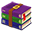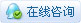533浏览

# [ESP8266/ESP32] FireBeetle 实现变幻线

 CRT(阴极射线显像管)显示器的显像原理主要是由灯丝加热阴极，阴极发射电子，然后在加速极电场的作用下，经聚焦极聚成很细的电子束，在阳极高压作用下，获得巨大的能量，以极高的速度去轰击荧光粉层。这些电子束轰击的目标就是荧光屏上的三原色。为此，电子枪发射的电子束不是一束，而是三束，它们分别受电脑显卡R、 G、 B三个基色视频信号电压的控制，去轰击各自的荧光粉单元，从而在显示屏上显示出完整的图像。在图形界面的操作系统下，显示屏上显示的色彩多种多样，当用户停止对电脑进行操作时，屏幕显示就会始终固定在同一个画面上，即电子束长期轰击荧光层的相同区域，长时间下去，会因为显示屏荧光层的疲劳效应导致屏幕老化，甚至是显像管被击穿。因此从Windows3.X时代至今，屏幕保护程序一直作为保护CRT显示屏的最佳帮手，通过不断变化的图形显示使荧光层上的固定点不会被长时间轰击，从而避免了屏幕的损坏。【参考1】比如，下面这个照片就是游戏《吃豆人》的烧屏，《吃豆人》历史久远，画面对比度高且单一，又是热门游戏，所以很多老机台烧屏都十分严重。【参考2】随着时代的发展，液晶屏成为了显示器的主流，屏保除了展示信息已经没有太多意义了（当然，它的存在还能保证当你休眠的时候不会有人手欠帮你按下关机）。Windows XP 开始，内置了一个非常经典的“变换线”屏保。这次的实验就是使用DFRobot 的FireBeetle通过VGA 接口实现这个功能。使用的硬件和库配置请参考之前的《FireBeetle 做一个 VGA 时钟》【参考3】一文：关键部分参考了《模拟经典屏保“变幻线”》【参考4】，主要是绘制直线。代码如下： .hljs {margin: 10px 0px;}.hljs ol,.hljs li{list-style: decimal;}.hljs li {margin-left: 0em;}``````#include "fabgl.h" // VGA 显示 fabgl::VGA16Controller DisplayController; Canvas cv(&DisplayController); #define HIGH 480 #define WIDTH 640 #define RADIUS 20 #define PN 5 typedef struct {   int x;   int y;   int velocity_x;   int velocity_y; } VPOINT; static VPOINT vpoint[PN]; void setup() {   Serial.begin(115200);   DisplayController.begin();   // 设定分辨率   DisplayController.setResolution(VGA_640x480_60Hz);   int             a;   cv.setPenColor(0xFF,0,0);   for (int i = 0; i < PN; i++)   {     vpoint[i].x = rand() % (WIDTH - RADIUS) + RADIUS;     vpoint[i].y = rand() % (HIGH - RADIUS) + RADIUS;     a = rand() % 361;     vpoint[i].velocity_x = (int)((RADIUS / 5) * cos(a));     vpoint[i].velocity_y = (int)((RADIUS / 5) * sin(a));   }   for (int i = 0; i < PN - 1; i++)   {     cv.drawLine(vpoint[i].x, vpoint[i].y,vpoint[i + 1].x, vpoint[i + 1].y);   }   cv.drawLine(vpoint.x, vpoint.y, vpoint[PN - 1].x, vpoint[PN - 1].y); } void loop() {   cv.clear();   for (int i = 0; i < PN; i++)   {     if ((vpoint[i].x <= 0) || (vpoint[i].x >= WIDTH))       vpoint[i].velocity_x = -vpoint[i].velocity_x;     if ((vpoint[i].y  <= 0) || (vpoint[i].y  >= HIGH))       vpoint[i].velocity_y = -vpoint[i].velocity_y;     vpoint[i].x += vpoint[i].velocity_x;     vpoint[i].y += vpoint[i].velocity_y;     for (int j = 0; j < PN - 1; j++)     {       cv.drawLine(vpoint[j].x, vpoint[j].y, vpoint[j + 1].x, vpoint[j + 1].y);     }     cv.drawLine(vpoint.x, vpoint.y, vpoint[PN - 1].x, vpoint[PN - 1].y);   } } 复制代码``````在电视机上的显示结果：完整代码：FB_SCLine.zip (906 Bytes, 下载次数: 26)### zoologist中级技匠 楼主|

 本帖最后由 zoologist 于 2022-1-7 21:23 编辑 工作的视频在:

 您需要登录后才可以回帖 登录 | 立即注册 本版积分规则 回帖并转播 回帖后跳转到最后一页

[[wsData.name]]

#### 硬件清单

• [[d.name]]#### 楼主的其它帖子